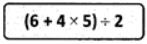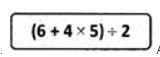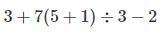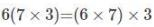Courses

# Important Questions Test: Whole Numbers

## 20 Questions MCQ Test Mathematics (Maths) Class 6 | Important Questions Test: Whole Numbers

Description
This mock test of Important Questions Test: Whole Numbers for Class 6 helps you for every Class 6 entrance exam. This contains 20 Multiple Choice Questions for Class 6 Important Questions Test: Whole Numbers (mcq) to study with solutions a complete question bank. The solved questions answers in this Important Questions Test: Whole Numbers quiz give you a good mix of easy questions and tough questions. Class 6 students definitely take this Important Questions Test: Whole Numbers exercise for a better result in the exam. You can find other Important Questions Test: Whole Numbers extra questions, long questions & short questions for Class 6 on EduRev as well by searching above.
QUESTION: 1

Solution:

4+4 - 3 = 5
or
8-3 = 5

QUESTION: 2

### Four students simplify the expression below.Anshu's solution is 25, David's solution is 46, Jimmy's solution is 13, and Lahari's solution is 16. Who simplified the expression correctly?

Solution:= ( 6 + 4 x 5) / 2

= (26) / 2  As BODMAS rule is applied and hence multiplication is done first and then 6 is added.

= 13

QUESTION: 3

### Simplify the expression below.Solution:By using BODMAS rule ,

= 3 + 7 (6)  ÷ 3 - 2

= 3 + 42  ÷  3 - 2

= 3  + 14 - 2

= 17 - 2

=  15

QUESTION: 4

Which is the whole number that does not have a predecessor?

Solution:

0 does not have a predecessor.

QUESTION: 5

Which of the following is a correct statement?

Solution:

Natural numbers are contained in whole numbers

QUESTION: 6

The product of the place values of two 2's in 428721 is

Solution:

The Number is 428721.
2 is in place of tenth and ten thousand.
Those two numbers are 20 and 20,000
So,
The product of those two numbers are :
=> 20 × 20,000
=> 4,00,000

QUESTION: 7

3 x 10000 + 7 x 1000 + 9 x 100 + 0 x10 + 4 is the same as

Solution:
QUESTION: 8

If 1 is added to the greatest 7- digit number, it will be equal to

Solution:

First, the largest 7 digit number is --?
Its 9,999,999
9,999,999 + 1 = 10,000,000
Or in Indian numbering system - 1,00,00,000

QUESTION: 9

When rounded off to nearest thousands, the number 85642 is

Solution:
QUESTION: 10is an example of which property with respect to multiplication?

Solution:
QUESTION: 11

The largest 4-digit number, using any one digit twice, from digits 5, 9, 2 and 6 is

Solution:
QUESTION: 12

In Indian System of Numeration, the number 58695376 is written as

Solution:

QUESTION: 13

The greatest number which on rounding off to nearest thousands gives 5000, is

Solution:

The greatest number which on rounding off to the nearest thousands gives 5000 is 5499.

QUESTION: 14

Keeping the place of 6 in the number 6350947 same, the smallest number obtained by rearranging other digits is

Solution:

Keeping 6 fixed at its own place. If we want to create a smallest number. Then we can do it by using the small numbers from the remaining numbers.
After 6, 0 will come, then 3, 4,5,7,9.
Therefore, option 'c' is the correct answer.

QUESTION: 15

What is the predecessor of natural number 1?

Solution:

There is no predecessor for natural number 1.

QUESTION: 16

The largest 5-digit number having three different digits is

Solution:

The largest 3 digits are: 9,8 and 7.

We have to form largest 5 digit number using 9,8 and 7.
Therefore, the largest 5- digit number having three different digits is  99,987

QUESTION: 17

The smallest 4-digit number having three different digits is

Solution:
QUESTION: 18

Number of whole numbers between 38 and 68 is

Solution:
QUESTION: 19

The product of successor and predecessor of 999 is

Solution:

Successor=1000
Predecessor=998
Product= 1000×998
= 998000

QUESTION: 20

A whole number is added to 25 and the same number is subtracted from 25. The sum of the resulting numbers is

Solution:

Let the whole number be x
According to the question
= x + 25 + 25 - x
= 50